Journal of Electrical and Electronic Engineering
Volume 4, Issue 3, June 2016, Pages: 78-82

A Robust and Adaptive Line Echo Canceller

Wenhong Liu

School of Electronic and Information, Shanghai Dianji University, Shanghai, China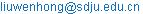Wenhong Liu. A Robust and Adaptive Line Echo Canceller. Journal of Electrical and Electronic Engineering. Vol. 4, No. 3, 2016, pp. 78-82. doi: 10.11648/j.jeee.20160403.17

Received: June 27, 2016; Accepted: July 14, 2016; Published: July 15, 2016

Abstract: In the actual communication system, the line echo is a frequent problem, so the line echo canceller is necessary. Traditionally, the cancellers are designed according to line noises based on the Gaussian noise model. However, the noises do not obey the Gaussian distribution; therefore the performances of algorithms decrease, even cannot be used. In order to solve this problem, this paper proposes a line echo canceller based on alpha stable distribution model. The Method in this line echo canceller has Robust and Adaptive. Alpha stable distribution modeling impulse noise, and under the rule of minimum average p norm, adaptive time delay estimator offsets the echo. This method can not only effectively offset the line echo with the Gaussian noise, but also to have the effect of good to echo with impulse noise. Theoretical analysis and computer simulation experiments show that it is very kind of the line echo canceller. It is robust to impulse noises and adaptive to echo cancellation.

Keywords: Communication System, Line Echo Canceller, Alpha Stable Distribution, Adaptive Time Delay Estimator, Minimum Average p Norm

Contents

1. Introduction

Echo generally refers to through other way different from the normal path and arrived at a given point on the signal. On this point, the signal has enough size and time delay, so that can detect it with the signal transmitted from the normal path into the difference between . Human auditory can sense certain strength, the voice of the delay of more than 50 ms echo. Voice communications is one of the most basic telecommunication businesses, voice communication problems often met in echo .

In traditional Public Switched Telephone Network (Public Switched Telephone Network, PSTN), because by 2/4 wire conversion in the circuit impedance with the Telephone terminal impedance cannot match exactly, by 2/4 wire conversion circuit of the input signal will be part of the leak in the output signal. The speaker's voice signal in 2/4 wire conversion circuit from sending channel leakage to receive channel, leading to the speaker  and hear your voice. In telephone network based on Internet (Voice over Internet Protocol, VoIP), line echo is also one of the main reasons for producing echo when Voice calls. On the phone to the computer, the computer to a telephone service, need VoIP phone connects to PSTN gateway, the other end connected to the Internet. Although produced in PSTN line echoes, but will also spread to IP telephony gateway. Thanks to the Internet voice transmission are the packet switching technology to achieve a kind of brand-new telecommunications business, transmission of voice signal passes through coding, compression, packaging and a series of processing, which not only cause echo path delay is larger, and the delay jitter is larger. Therefore, in the process of the voice transmission of Internet, even modest echo can also lead to a sharp decline in call quality . Therefore, in the voice communication system of echo cancellation issues must be considered in the design process.

Solving the problem of echo the earliest USES is to cut off the echo path echo suppression method, this method affect double-end take turns to speak, to be being washed out gradually. M.M.S on dui et al., using the digital signal processing technology is developed and a kind of adaptive echo canceller . At home and abroad for many years, the theory of adaptive echo cancellation and technology are studied, some key technologies in recent years, is one of the problems put forward some different algorithms [5-7], also obtained the actual application. Voice echoes in line, not just the pure speech signal, it is always mixed with noise. Many of the methods developed so far, the noise by using the Gaussian model to describe more, but a lot of practical study shows that the actual telecommunication lines in the system with pulse noise often [8,9], the classic algorithm based on Gaussian noise model is not robust, algorithm performance degradation, and can't even use [10-15].

In this paper, according to the characteristics of the noise in practical communication system, using Gaussian distribution than the more in conformity with the actual noise, stable and Robust α distribution to describe the noise, Echo in minimum average p Norm (further Mean p Norm, LMP) criterion, design a kind of impulse noise Robust Adaptive Line Echo Canceller (Robust Adaptive Line Echo Canceller, RALEC). This method can not only effectively offset line contains the echo of the Gaussian noise, but also to contain impulse noise echo offset the effect of good. Theoretical analysis and computer simulation experiment results show that the RALEC is a kind of the impulse noise robust adaptive echo cancellation method, has application value.

2. Alpha Stable Distribution and Minimum Average p Norm

Alpha stable distribution is a kind of generalized Gaussian distribution, the concept was first in 1925 by the Levy in the study of generalized central limit theorem is given, it is to meet the generalized central limit theorem is the only type of distribution, is a can keep natural limiting distribution of the noise generation mechanism and propagation conditions, can very well in conformity with the actual data. Alpha stable distribution for many phenomena in different areas provides a very useful model, should have applications in physics, electronic information engineering, hydrology, biology, and economics. In the field of signal processing and communication, α stable distribution also got many important applications. From a different angle, we can give the definition of several kinds of α stable distribution . The probability density function is commonly used in signal processing, for α stable distribution, existence and continuous, but in addition to a few, were not analytic expression, but many use probability density function of the work can be done through transformation of characteristic function, therefore, α stable distribution is usually use the following function to describe the characteristics of a :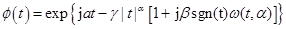(1)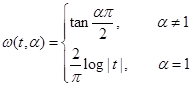(2)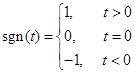(3)

The omega (t, α) = tan (α PI / 2), α indicates 1; Omega (t, α) = (2 / PI) lg | t |, α = 1. α (0 < α 2 or less) as the characteristic index, determine the thickness of the probability density function is trailing, α value is smaller, its tail is thick; Beta (-1 < =beta< = 1) for the symmetric parameters, determine the degree of probability density function of deflection, when beta = 0, called symmetric α stable distribution, remember to S α S; Gamma (gamma > 0) as the dispersion coefficient, a measure of the dispersion degree sample; Mu (- up < mu < + up) as the location parameters, for S α S distribution, distribution of average or median mu said.

Alpha stable distribution function of the four parameters, is the most important characteristic index of α, its value is smaller, the statistical distribution away from the more sample mean or the median, the pulse of the more significant in the time domain waveform signal. When 0 < α < 2, called fractional lower order α stable distribution (fractional lower order α - stable distribution, FLOA); When α = 2 S α S is Gaussian distribution, therefore, Alpha stable distribution is a kind of generalized Gaussian distribution, the distribution of the Gaussian distribution for α stability a special case. Research shows that when the noise is pulse is not in conformity with the Gaussian distribution, based on the stability of α than algorithm based on Gaussian distribution assumption assumes that the algorithm has robustness; When the noise is Gaussian distribution, the performance of the algorithm and the latter also quite .

Under Gaussian assumption, usually using second order statistics as the optimal criterion of signal analysis and processing, such as least square criteria and minimum mean square error criterion and so on. But in FLOA assumption that there is no signal or noise second order or higher order moment, based on second order statistics of the optimal criterion cannot achieve the optimal. Due to dispersion coefficient of α stable distribution of gamma measurement of the sample dispersion degree, based on the stability of α distribution process of linear theory, the minimum mean square error criterion is to promote as minimum dispersion coefficient criterion. Through minimize estimate error of dispersion coefficient, average amplitude estimation error can be implemented to minimize. Estimation error is equivalent to minimize the dispersion coefficient of the estimation error of the fractional lower order moment - Lp minimizing, Lp norm have excellent ability to inhibit the spike pulse [16-19]. As a result, the algorithm based on α stable distribution model, the LMP is a commonly used robust optimization criteria [20-21].

3. Robust and Adaptive Echo Canceller

Echo offset method the basic idea is to speakers normal speech signal after a delay, rather than line echo signal subtraction, so as to eliminate echo on the speaker answered. Echo offset, therefore, the core of the problem is the time delay estimation, namely line echo time estimates. RALEC method proposed in this paper is essentially based on robust adaptive time delay estimation of echo offset and its principle block diagram is shown in figure 1.Fig. 1. Block diagram of the echo cancellation -based on robust adaptive TDE.

Figure 1 is based on the robustness of adaptive time delay estimation echo offset principle block diagram. In echo cancellation problem as above, can assume that contain line noise speech signal and the echo signal of x1 and x2 (n) (n) meet the following discrete signal model: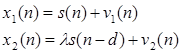(4)

s(n) for speech signal from the speaker, s (n - d) as the speaker voice signals in the talent exchange center line transformation of echo signal; D for echo signal delay time; Lambda for echo signal attenuation factor (because of the performance of the proposed algorithm is not affected by the influence of the parameters, easy to often take lambda = 1); v1, v2 (n) (n) respectively for speech signal and echo signal contains noise, α stable distribution is used to describe. Usually, we can assume that signal and noise, noise and noise are statistically independent.

In the RALEC method, adaptive time delay estimator is the core part of the echo canceller, it is used to produce a similar simulation and circuit echo. Real echoes and simulation after subtracting, can greatly reduce the echo level on return to talk. Adaptive time delay estimator is by the input signal, adaptive filter, the reference signal, subtracted, optimization criteria. Input signal for speaker from the speech signal with noise, the x1 (n), the reference signal for the echo signal contains noise line x2 (n), filter using moving average MA filter, optimization guidelines adopted the LMP.

MA moving average filters to simulate the communication line for delayed effect of speech signals x1 (n):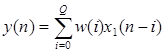(5)

W (I) (I = 1 ~ Q) is the MA model parameter, Q > d. At I = d w (I) have a maximum value, namely I d | w (I) | < (d) | | w.

Equator (5) can be written as the following form of the vector: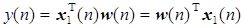(6)

Error signal that residual echo e (n)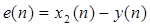(7)

RALEC algorithm of the cost function J (w)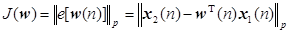(8)

Using the steepest descent method and the cost function J (w), the smallest value of filter weight w (n) for iterative equation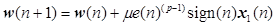(9)

Equator (9), convergence factor for the adaptive filter, the LMP convergence condition of the adaptive filter, adaptive filter Q + 1 said the number of weights, for input signal power. Weight coefficient of the convergence rate and is proportional to the, in the case of satisfy the convergence condition, the greater the, system the faster convergence. But the larger convergence factor can cause larger weight coefficient estimation error, couldn't even make the algorithm convergence. Therefore, needs to consider the convergence speed and estimation precision, two factors made compromise between them.

Estimate of the echo signal delay time is essentially the weight coefficient of the MA filter estimate maximum position. In the process of RAEC adaptive iteration algorithm, search step by step, when the right of adaptive filter coefficient convergence, y (n) and x2 (n) to minimize the average error between p norm, namely the y (n) similarity with x2 (n) is the largest, at this point, the subtracted output echo minimum residual signal, achieve the goal of the echo offset.

The computer simulation experiment and the results as followed:

Based on MATLAB software, the computer simulations are made validation of RALEC algorithm under the condition of Gaussian and non-Gaussian noise estimation performance, and with the AEC algorithm under the condition of two kinds of noise.

According to (4) two lines of received signal in structure, including voice signal s (n) for band-limited flat spectrum signal, noise v1, v2 (n) (n) with α to simulate the stable distribution. Mixed signal-to-noise ratio according to the MSNR = 10 lg (sigma s2 / γ v) setting, the sigma s2 represents the source signal variance, γ the dispersion coefficient of v noise, Gaussian noise, dispersion coefficient is half of the variance. Set the line echo signal delay time d = 20. Signal sequence of length n = 5000 points, selection of MA order number m = 25 of the filter, filter weight vector of the initial value setting of w (0) = 0] [0... RALEC and AEC algorithm of iterative step are mu = 0.0001. The following are the result of the 100 independent experiment statistics.

First, under the condition of Gaussian noise, α = 2, MSNR = 0 db, the AEC and RAEC algorithm is adopted to set the line echo signal delay time estimate d, observe two algorithm convergence curve, comparison of the estimated performance of the algorithm.

Figure 2 AEC algorithm in α = 2, under the condition of MSNR = -3 db, right of convergence when the filter coefficient curve (a) (b) echo delay time convergence curves. The result of the AEC algorithm is shown in figure 2.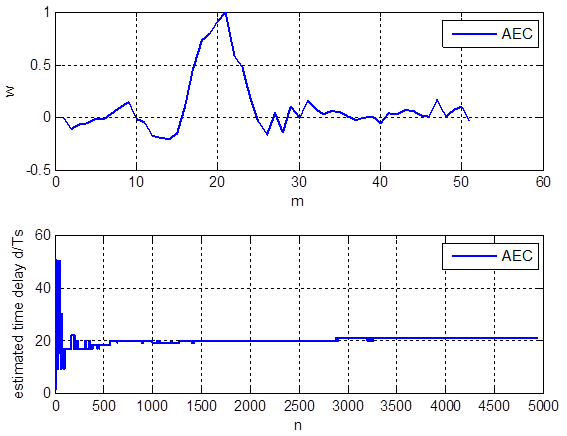Fig. 2. AEC algorithms with α = 2 and MSNR = -3 db (a) weight efficiency curve under convergence condition (b) convergence curve of estimated echo delay time.

In α = 2, figure 3 RALEC algorithm, under the condition of MSNR = -3 db (a) right of convergence when the filter coefficient curve (b) echo delay time convergence curves. The result of the RALEC algorithm is shown in figure 3.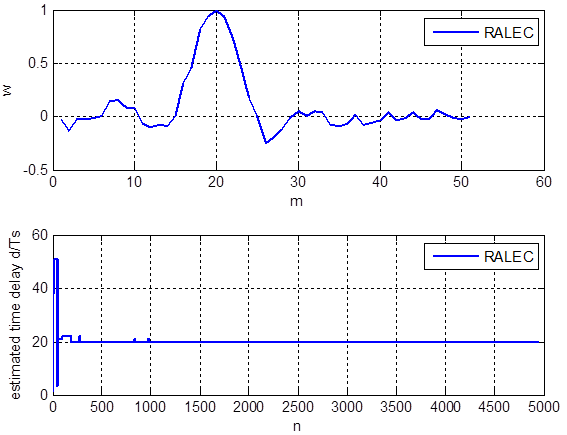Fig. 3. RALEC algorithms with α = 2 and MSNR = -3 db (a) weight efficiency curve under convergence condition (b) convergence curve of estimated echo delay time.

As you can see from figure 2 and figure 3, in α = 2, under the condition of MSNR = -3 db, AEC and RALEC algorithm for echo delay time estimates are accurate, that under the condition of Gaussian noise, estimate the performance of both methods.

Further, under the condition of non Gaussian pulse noise, α = 1.6, MSNR = -3 db, the AEC and RALEC algorithm is adopted to set the line echo signal delay time estimate d, observe two algorithm convergence curve, comparison of the estimated performance of the algorithm.

Figure 4 AEC algorithm in α = 1.6, under the condition of MSNR = -3 db, right of convergence when the filter coefficient curve (a) (b) echo delay time convergence curves. The result of the AEC algorithm is shown in figure 4.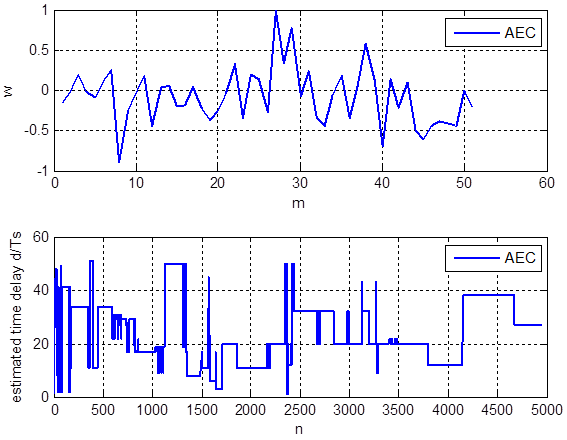Fig. 4. AEC algorithms with α = 1.6 and MSNR = -3 db (a) weight efficiency curve under convergence condition (b) convergence curve of estimated echo delay time

Figure 5 RALEC algorithm in α = 1.6, under the condition of MSNR = -3 db, right of convergence when the filter coefficient curve (a) (b) echo delay time convergence curves. The result of the RALEC algorithm is shown in figure 5.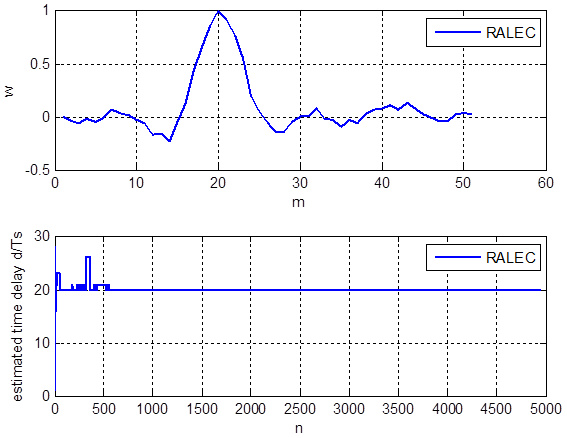Fig. 5. RALEC algorithms with α = 1.6 and MSNR = -3 db (a) weight efficiency curve under convergence condition (b) convergence curve of estimated echo delay time.

As you can see in figure 4 and 5, in α = 1.6, under the condition of MSNR = -3 db, AEC algorithm can't convergence, therefore, not out of the echo signal delay time estimates, while the RALEC algorithm convergence in iteration after 2000 points, can be set to estimate the true value of d = 10.

More than the computer simulation results show that the AEC algorithm applies only to Gaussian noise environment; RALEC algorithm suitable for the Gaussian and non-Gaussian impulsive noise, therefore, for the impulse noise which has better robustness than the former.

4. Conclusion

In the practice, there are impulsive noises with communication lines. This makes the traditional adaptive echo cancellation methods become poor, noises model based on Gaussian distribution. To solve the problem, this paper presents a robust adaptive echo cancellation method based on α stable distribution, referred to RALEC. RALEC describes impulse noise by Alpha stable distribution. Under the least mean p norm criterion, the echo is cancelled by using adaptive time delay estimator. This method can not only effectively offset the echo of communication lines in the presence of Gaussian noises, but also have good offset effect in the presence of non-Gauss. Echo offset problems in communication lines, core technologies is estimate echo delay time. Estimate echo delay time is essentially a time delay estimation problem. Therefore, the research of this paper is time delay estimation theory and technology in an application example in the field of communications. RALEC is a kind of based on robust adaptive time delay estimation of echo cancellation method. The method MA filter is adopted to form the core part of the time delay estimator, to simulate the line echo effect, under the rule of LMP, achieve the optimal. RALEC can not only effectively offset line contains the echo of the Gaussian noise, but also to contain impulse noise echo offset the effect of good, of impulse noise is a kind of robust adaptive echo cancellation method.

Acknowledgements

This work was supported by 2016 Shanghai Dianji University Leading Courses Construction on Signals and Systems,2016 Shanghai Dianji University Leading Teaching Research and Reform Projects on Reform and Practice on the Teaching of Professional Basic Course Group in the Wide Field, and Shanghai Dianji University Leading Discipline on Computer Application Technology with project No.13XKJ01. I would like to be very grateful to my colleagues, Lecture Fan Guangyu, Prof. Chen Niansheng, and Lecture Liu Zhonghua, and my doctoral advisor Prof. Qiu Tianshuang in Dalian University of Technology.

References

1. http://shuyu.cnki.net/SearchResult.aspx?sItem=%u56DE%u6CE2&ids, 2012.3. Communication Science and Technology Terminology Committee. Communications Science and Technology Terms (definition of edition).
2. Song Aimin. New Algorithms for Time Delay Estimation and Beam forming under Stable Distribution Noise [D]. Dalian University of Technology, 2015.
3. Cai Ping. Research on Algorithms of Adaptive Echo Cancellation [D], [Dissertation]. Southeast University, 2007.
4. Bao Weihua. Study on Echo cancellation technique in the environment of mobile communication [D]. [Dissertation], Heibei University, 2014.
5. Nikiasc l, Shao M. Signal Processing with Alpha-stable Distributions [M]. New York: John Wiley & Sons Inc, 1995.
6. Bao Weihua. Study on Echo Cancellation Technique in the environment of mobile communication [D]. Hebei University, 2014.
7. Wada, Ted S. Enhancement of residual echo for robust acoustic echo cancellation [J]. IEEE Transactions on Audio, Speech and Language Processing, 2012, 20(1): 163-177.
8. Sondhi M M. An adaptive echo canceller [J]. Bell syst. Tech. J., 1967, 46.
9. Shimauchi, Suehiro, Haneda, et al.. Frequency domain adaptive algorithm with nonlinear function of error-to-reference ratio for double-talk robust echo cancellation [J]. Acoustical Science and Technology, 2005, 26(1): 8-15.
10. Rajan, Adithya, Tepedelenlioglu, Cihan. Diversity combining over Rayleigh fading channels with symmetric alpha-stable noise [J]. IEEE Transactions on Wireless Communications, 2010, 9(9): 2968-2976.
11. TangHong, Li Ting, Qiu, Tian-shuang, et al. Constant modulus algorithm for cochannel signal separation in non-gaussian impulsive noise environments [J]. Wireless Personal Communications, 2010, 54(4): 591-604.
12. Liu Wen-hong. Robust Time Delay Estimation and Application in Evoked Potential Latency Delay Detection [D]. [Dissertation], Fudan University, 2009.
13. Ge F X, Shen D X, Peng Y N, et al. Super-resolution time delay estimation in multipath environments. IEEE Wireless Communications and Networking Conference, 2004, 2: 1121-1126.
14. Zhang Jinfeng, Qiu Tianshuang, Li Sen. A Robust PAST Algorithm Based on Maximum Correntropy Criterion for Impulsive Noise Environments [J]. ACTA ELECTRONICA SINICA. 2015, 43(3): 483-488.
15. Garel B, d’Estampes L, Tjostheim. Revealing some unexpected dependence properties of linear combinations stable random variables using symmetric covariation. Communication Statistics Theory and Methods 2004, 33(4): 769-786.
16. Ludeman L C. Random processes: filtering, estimation, and detection. New York: John Wiley and Sons, Inc., 2004.
17. Li Huiya, Yang Jinpo, Yin Yongchao, et al. Echo cancellation based on additional signal [J]. Journal Computer Application. 2013, 33(3): 631-634.
18. Gunther, Jake. Learning echo paths during continuous double-talk using semi-blind source separation [J]. IEEE Transactions on Audio, Speech and Language Processing, 2012, 20(2): 646-660.
19. Zhu You-1ian, Lu You-hong, Shi Guo-dong.  New method for adaptive Echo cancellation in communication systems [J]. Journal on Communications, 2007, 28(5): 67-72.
20. Li Jingwei, Qiu Houde. Correntropy Method for Spectrum Estimation in Impulsive Noise Environment [J]. Journal of Signal Processing, 2014, 30(8): 944-948.
21. Kidmose P. Adaptive filtering for non-Gaussian processes. IEEE ICASSP, 2000, 1: 424-427.

 Contents 1. 2. 3. 4.
Article ToolsAbstractPDF(213K)